# LOG#082. Group Theory (II).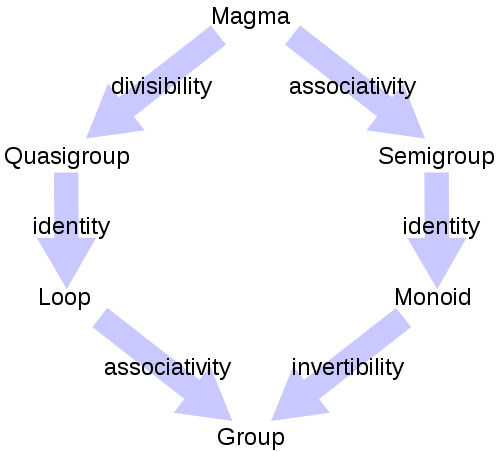Basic definitions of group theory: that is the topic today! We need some background previous to the “group axioms”.

Definition (1). Set is a collection of objects with some properties. Objects in the set are called “elements” or “members” of the set.

Definition (2). Category is some collection of objects linked or related theirselves with “arrows” or “maps”. Example: set is a category formed with sets as objects and functions as arrows. Other categories are, e.g., Top (topological spaces) or Ring (ring structures).

Category theory studies the main mathematical entities and their relationships, and it is a branch of mathematics which seeks to generalize all of mathematics in terms of objects and arrows, independent of what the objects and arrows represent. Virtually every branch of modern mathematics can be described in terms of categories, and doing so often reveals deep insights and similarities between seemingly different areas of mathematics. The nice fact about “group theory” is that there is a complete list of “group-like” structures: magma, semigroup, monoid, group, abelian group, loop, quasigroup, groupoid, category, semicategory, vector space, ring, semi-ring, and many other cool structures.

Definition (3). Function f is every map/relation/application between two sets X (domain) and Y(codomain) which associates each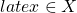with exactly one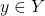, and it is usally denoted by. Mathematically speaking:Defintion (4). Functor F is every morphism/map/application of any category A to another category B.Definition (5). Binary operation. Let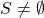be a set. A binary operationis any function taking “pairs” or “couples” of elements from that set into the own set. Mathematically speaking: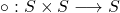and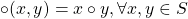Examples of binary operations are the usual operations of addition(+), substraction(-), product(x) and division(/) of real numbers.

Definition (6). Given a set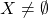, a binary relation R in X is a subset. Usually we writeinstead of the confusing notation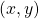.

Definition (7). A binary relation R inis called an equivalence relation if it satisfies the following conditions:

1) Reflexitivity:.

2) Symmetry: Ifthen,.

3) Transitivity: Ifand, then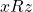,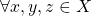.

This gadget, the equivalence relation, allow us to classify the elements of every set in disjoint classes: the so-called equivalence classes.

Definition (8). Equivalence class. Letbe an equivalence realtion,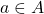is its equivalence class, denoted by, whenever we define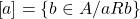We are ready for studying the group axioms right now!

Definition (9). Group is a pairwhere G is a non empty set (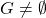) andis an internal, binary operation in G that satisfy 3 simple properties:

1. Associativity: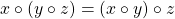,.

2. Identity/neutral element (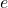):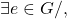it satisfies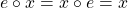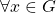.

3. Invertibility/inverse element (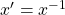):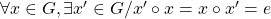.

That is all folks! Well, not exactly…There are other complementary concepts we can define:

Definition (10). Abelian group. An abelian group is any groupwith satisfy the commutativity property for its binary/product operation. That is, an abelian group satisfy that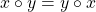Otherwise, the group is said to be non-abelian. Groups are generally non-abelian but there are some important abelian groups.

Definition (11). Semigroup. Letbe a set, and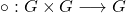a binary operation that satisfies tha associative property. Then the pairis called a semigroup. That is, a semigroup is an associative binary operation but without the identity or inverse properties that groups have!

Definition (12). Quasigroup.  A quasigroup is a pairsuch asis a set and the binary operationsatisfies the quasigroup law/property: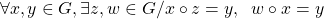Definition (13). Order. The order of a groupis the cardinal or number of elements in the structure.

Generally, the order of a group is denoted between vertical bars,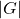or by.

Ifis a group, then its elements satisfy the following rules that can be derived from the definitions above in a trivial fashion:

1) The identity element, or neutral element, in a group is unique.

Check: Ifis another identity element, then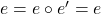.

2) In a group G, if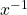is the inverse ofthenis the only inverse for that element.

The proof is equivalent to the previous one. It is let as an exercise for the reader.

3)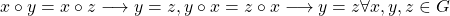.

4), and where the prime denotes the inverse element.

5)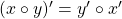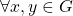.

6).

7)is abelian if and only if (iff).

See you in the next group theory blog post!

This site uses Akismet to reduce spam. Learn how your comment data is processed.